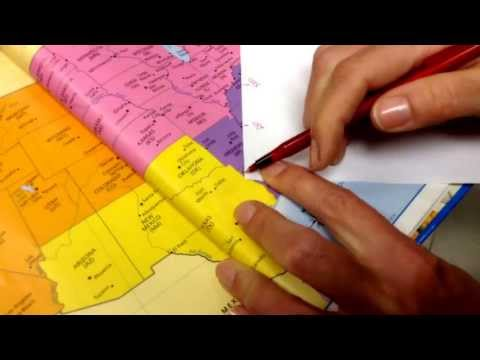## Knowledge on Distance

Subject: Social Studies

#### Overview

Statement scale is the method of expressing the ratio of length on paper and on the surface in words. The fractional method for portraying the scale of a map uses a representative fraction to describe the ratio between the map, and the real world is representative scale. The depicts scale using a line with separation mark by smaller intersection lines, smaller to a ruler is Graphical scale.
##### Knowledge on Distance

It is so difficult to show the actual shape and size of an object on a page of the paper. So, it should be shown using the scale. Obviously, this helps greatly to find out the actual shape and size of an object. In the map, one metre can be represented by one centimetre. The scale can be expressed in three different ways :-

1. Statement scale
It is also called verbal scale. It is the method of expressing the ratio of length on paper and on the surface in words. For example'one inch equals to one hundred and sixty miles.'

2. Representative scale
The fractional method for portraying the scale of a map uses a representative fraction to describe the ratio between the map, and the real world. For example - 1:50,000 or 1/50,000. It means 1 unit of distance on the map means 50,000 of the same unit of distance in the real world.

3. Graphical scale
It depicts scale using a line with separation mark by smaller intersection lines, smaller to a ruler.
The above given map show asmall portion of Pokhara valley. There are the places of Pokhara and Palpa area. If we use the greater scale and observe closely scale than this, we can show Ranimahal. We need to use the suitable scale depending upon the necessity. There is a specific objective of drawing a map that determinesa map that determines the type of scale to be used
.
##### Things to remember
• Statement scale is also called verbal scale.
• It includes every relationship which established among the people.
• There can be more than one community in a society. Community smaller than society.
• It is a network of social relationships which cannot see or touched.
• common interests and common objectives are not necessary for society.
##### Videos for Knowledge on Distance##### Questions and Answers

If one centimeter equals to one kilometer is to be shown in the fraction, it will be 1:100000. So, if the theory one centimeter equals to five kilometer is to be changed into fraction, there will be 1:5,00000.

The area of my house is:

Length of my house (l) = 20m

Width of my house (b) = 5m

The area of my house (A) = ?

A= l x b= 20 x 5 = 100m2.

Therefore, the area of my house is 100m2.

Map is the representation of whole or some parts on the earth or any other parts, or objects found in any body of universe on a flat sheet of paper with appropriate scale and color (so that each of the point in the map can show the real difference in location of any objects).

There is no field of study where there is no use for map. Map expresses a lot of spatial information through graphics that is easy to extract and understand. It’s relationship in combination with social attributes. Moreover, it helps to understand the problems and prospects of an area in terms of resources, infrastructures, etc. maps present multiple layers of information simultaneously; so we can compare different variables quickly. That is why it is said that picture is worth thousand words.